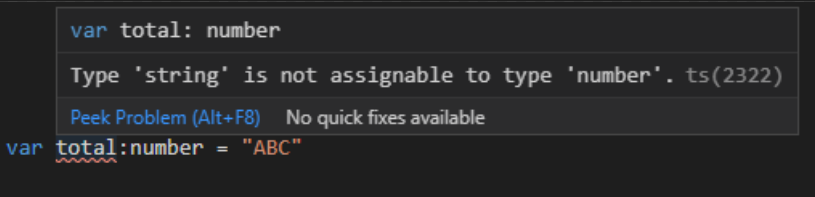Skip navigation

# Typescript, explained.

Published 2021/05/12 14:00

What is TypeScript? You've heard about it as the perfect companion to Javascript. But what is it and how does it work?

## Let's explain!

Take a look at this Javascript code:

``````var number1 = 5
var number2 = 10

var sum = number1 + number2

console.log(sum)
``````

What is the output of the code?

If your answer is 15 then you're right! You have two numbers, `number1` and `number2`. If you add them together using the `+` sign, it will give you the total because they're both numbers!

Now take a look at this:

``````var number1 = "5"
var number2 = "10"

var sum = number1 + number2

console.log(sum)
``````

This time `number1` and `number2` are strings. So what will this code give as output?

If your answer is `"510"` as a string, then you're right again!

But that's not how you expect it to work.

I want `number1`, `number2`, and `sum` to all be numbers so that `sum` gives me the actual sum every time.

Wouldn't it be nice if we could put some rules in so that we're absolutely CERTAIN that `number1`, `number2` and `sum` are ALWAYS numbers?

Let's look at another example:

Suppose, this time, we want to divide two numbers together:

``````var number1 = 10
var number2 = 2

var result = number1 / number2

console.log(result)
``````

The result of the above is (as you'd expect), `5`. But what if instead of a number, `number2` is a string, or an array?

``````var number1 = 10
var number2 = []

var result = number1 / number2

console.log(result)
``````

What would you expect it to happen here?

If your answer is "it would give me an error" then you'd be wrong. The result would be "Infinity". But that is non-sense!

There should be some rule in place that says "you can only divide numbers with numbers". Wouldn't that be nice?

Enter TypeScript!TypeScript is a language that is basically extended Javascript.

In essence, TypeScript allows you to write Javascript so that when you declare variables or functions, you can also specify what type each of them are (string, number, array etc).

For example:

"I want to create a variable called `total` which is a number":

``````var total:number = 5
``````

"I want to create a variable `name` which is a string":

``````var name:string = "Savvas"
``````

"I want to create a function `getTotal` which should always get a number array as input and always return a number":

``````function getTotal(values:number[]):number{
return values.reduce((accumulator, currentValue) => {
accumulator + currentValue
})
}
``````

So what would happen if I write a string in the total variable in my TypeScript (.ts) file, since it expects a number?

``````var total:number = "ABC"
``````

This:This is what's so amazing about TypeScript. It tells you that what you're assigning is wrong before you even hit save! That means this error never makes it to your users, and it makes fixing errors a lot LOT easier!

## So how do I get started?

You can install TypeScript using via NPM:

``````npm install -g typescript
``````

You can then turn a TypeScript (`.ts`) file into a Javascript (`.js`) file by running this command:

``````npx tsc myfile.ts
``````

You can then attach your JS file to your HTML as normal!

This thread only touches the absolute basics of TypeScript but you can learn a lot more on the TypeScript official documentation which is quite comprehensive!

Enjoy!

## Subscribe

Get the latest posts weekly straight to your inbox!